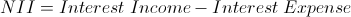Net Interest Income

Net Interest Income Calculator (Click Here or Scroll Down)The net interest income formula is used to calculate the amount of interest income that is left after covering interest expenses. The total interest income, total interest expense, and net interest income can be found on a bank's income statement.

Banks earn interest through loans, mortgages, and other similar interest earning products. On the other side is a bank's interest expenses. Examples of interest expenses for banks would be deposit accounts, such as CD's and savings accounts, along with other forms of debt the bank may hold.

Banks also have income and expenses that are unrelated to interest, and aren't included in this formula.

Example of Net Interest Income Formula

An example of the net interest income formula would be to suppose that a bank shows a net interest income of \$4 million on its statement of income. The information is already calculated. The variables, interest income and interest expenses, can also be found on the income statement. But let us assume that there was a change between this year and last year. Although the information is readily available, perhaps an individual would like to break down what specifically changed. Understanding the net interest income formula helps with this.

Use of Net Interest Income Formula

Net interest income can be used internally by banks along with other formulas to review the company's ability to earn profits. Investors who are reviewing a bank's financials may also be interested in this formula.

Additional formulas may be used as the net interest income alone doesn't offer much. Although this is an important part of a bank's operation, banks do make additional income from fees and services unrelated to interest. Banks also incur additional expenses unrelated to interest.

Aside from reviewing a particular company, this formula can also be used for overall review of the entire banking industry. A person reviewing the economy may take a look at the interest income for the economy as a whole.

The net interest income for commercial banks in the US can be found at the Federal Reserve Bank of St Louis: https://fred.stlouisfed.org/series/USNII.
They also have information for additional countries and each individual country should have this information available by that country's central bank.

New to Finance?